# NCERT Solutions Class 10 Science Chapter 12 Electricity

Here you can get free NCERT Solutions for Class 10 Science, of Chapter 12 Electricity. All NCERT Book Solutions are given here exercise wise for Electricity. NCERT Solutions are helpful in the preparation of several school level, graduate and undergraduate level competitive exams. Practicing questions from NCERT Science Book, its Solutions for Class 10 Chapter 12 Electricity is proven to enhance your subject skills.

 Class: 10th Class Chapter: Chapter 12 Name: Electricity Subject: Science

## NCERT Solutions Class 10 Science Chapter 12 – Electricity

NCERT Class 10 Solutions Chapter 12 – Electricity are given below.

NCERT Solutions for Class 10 Science Chapter 12 Electricity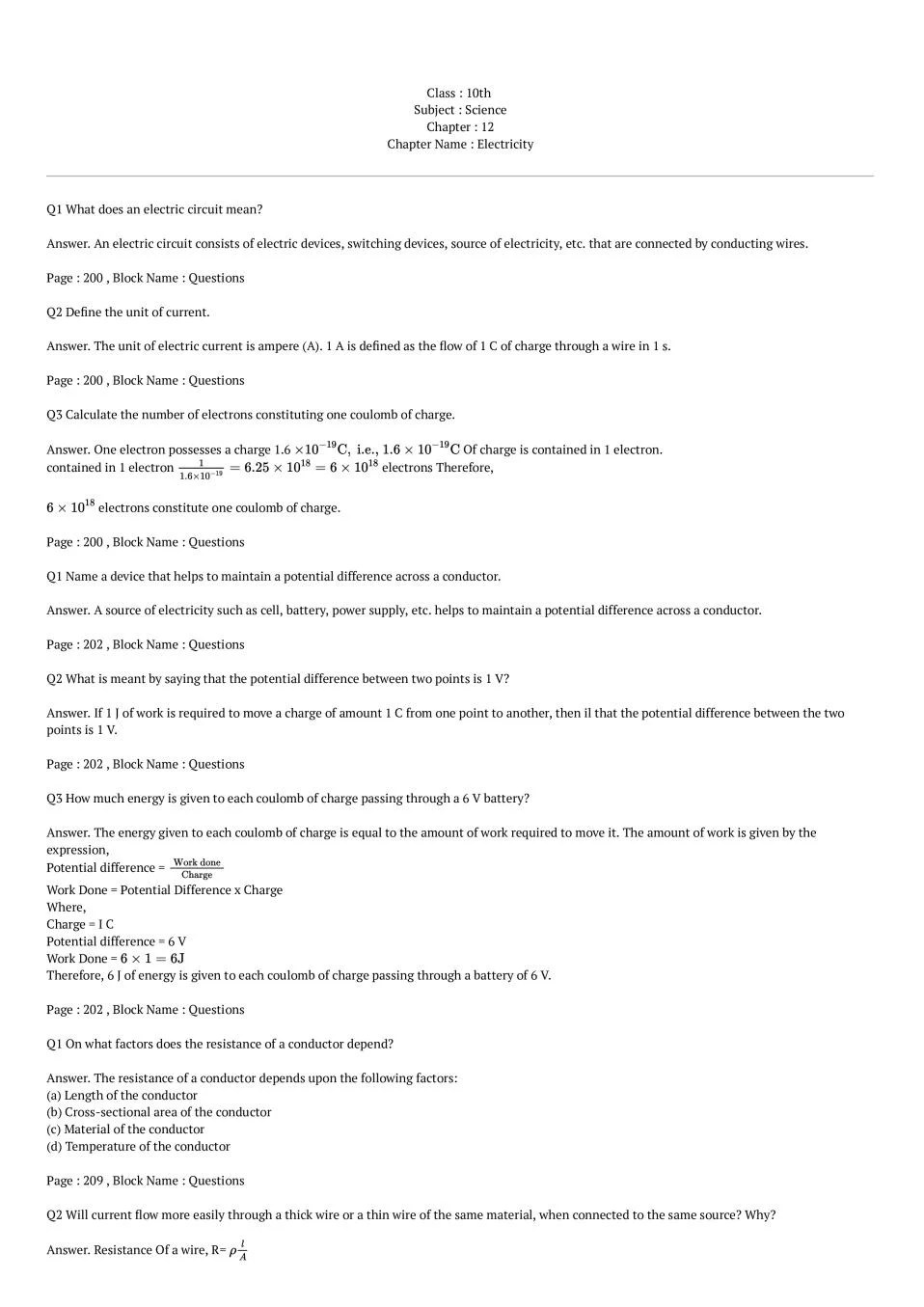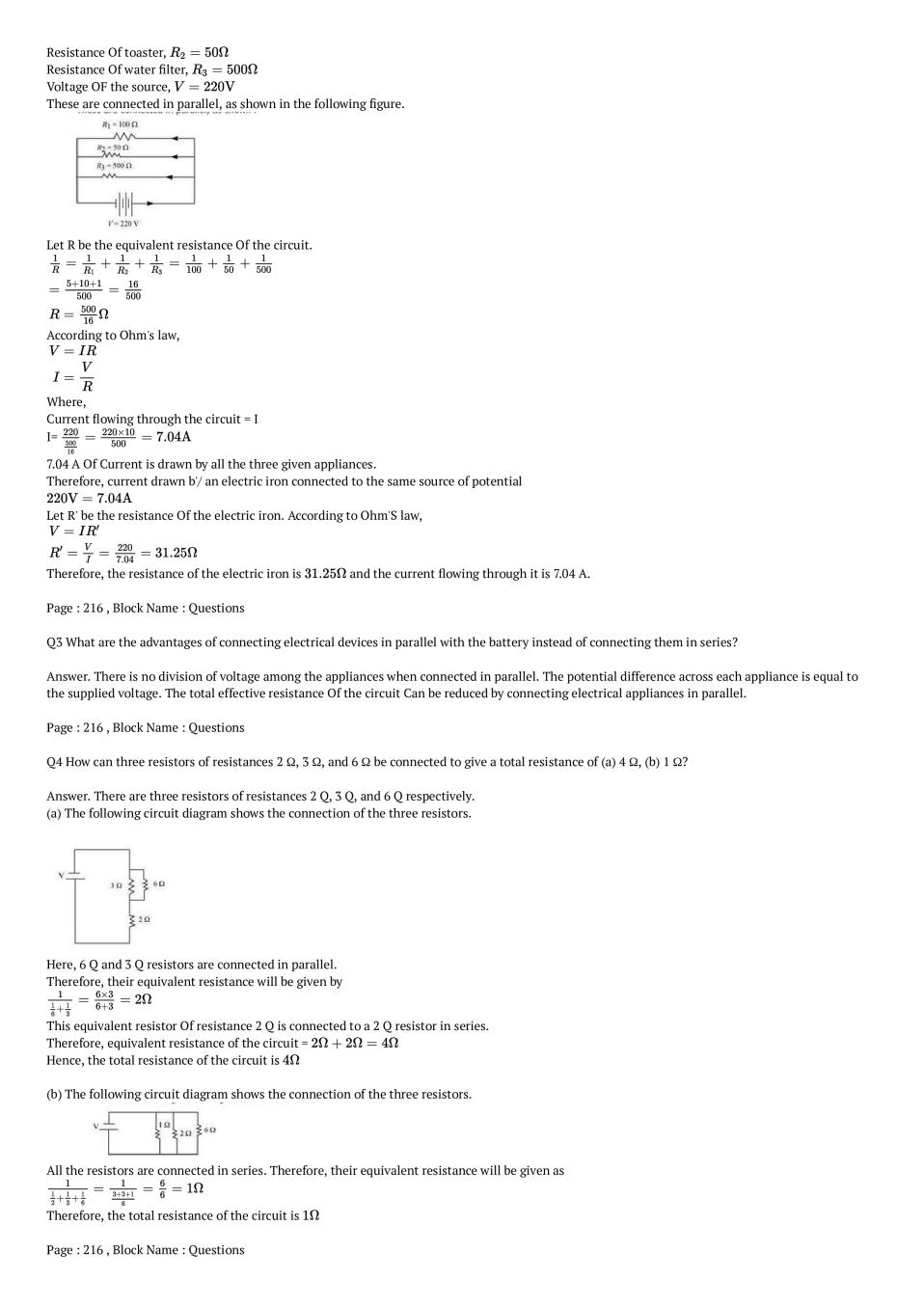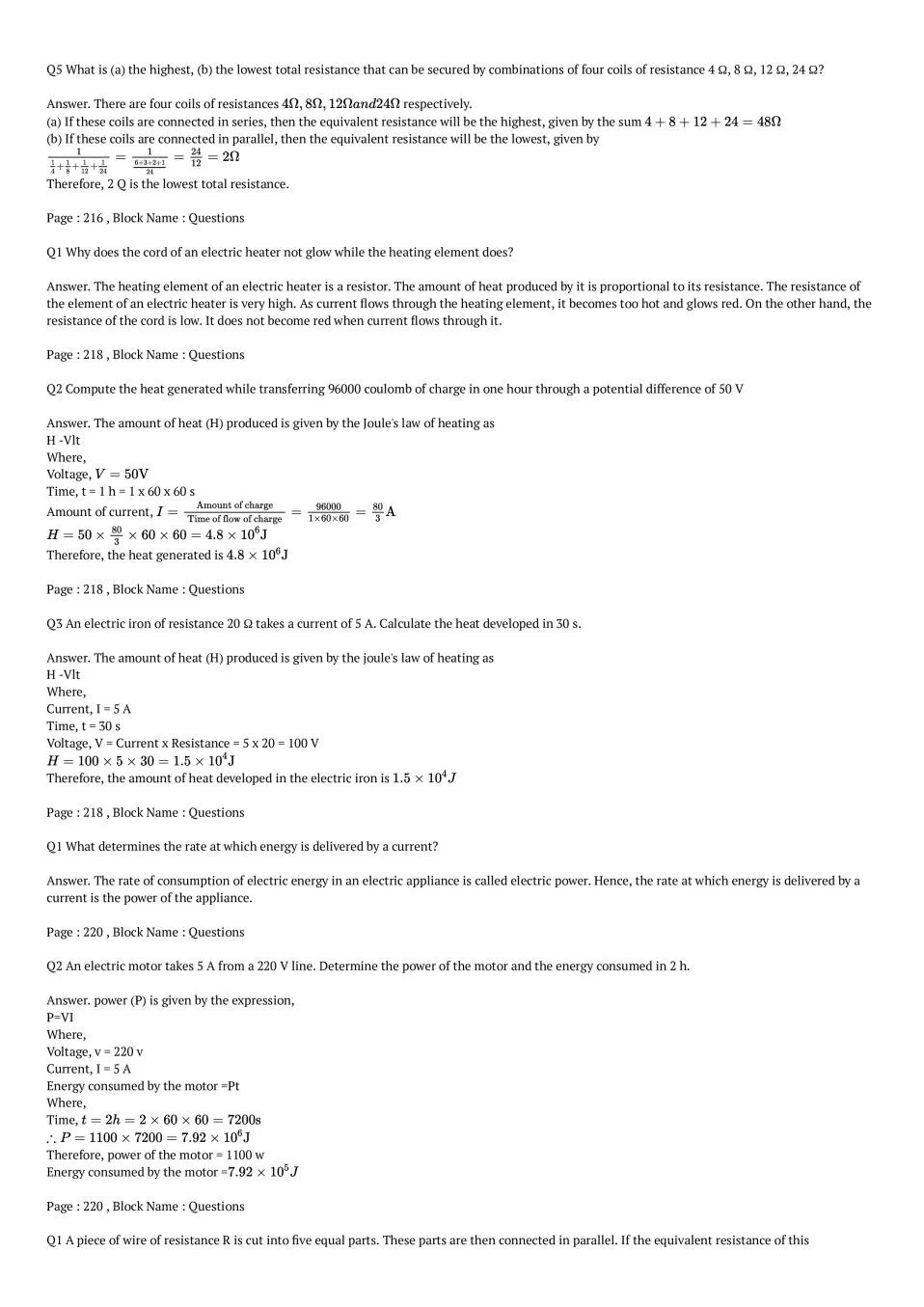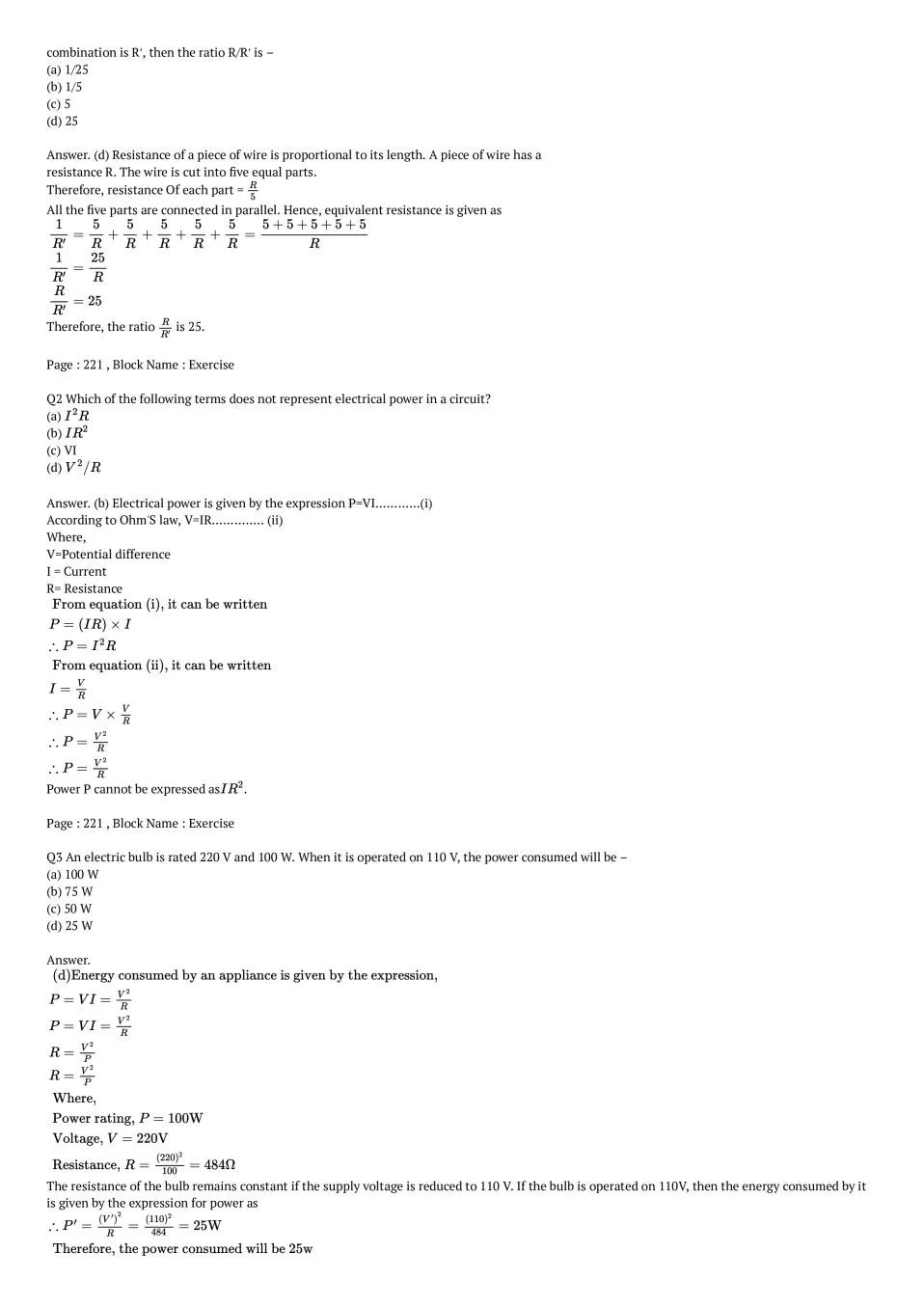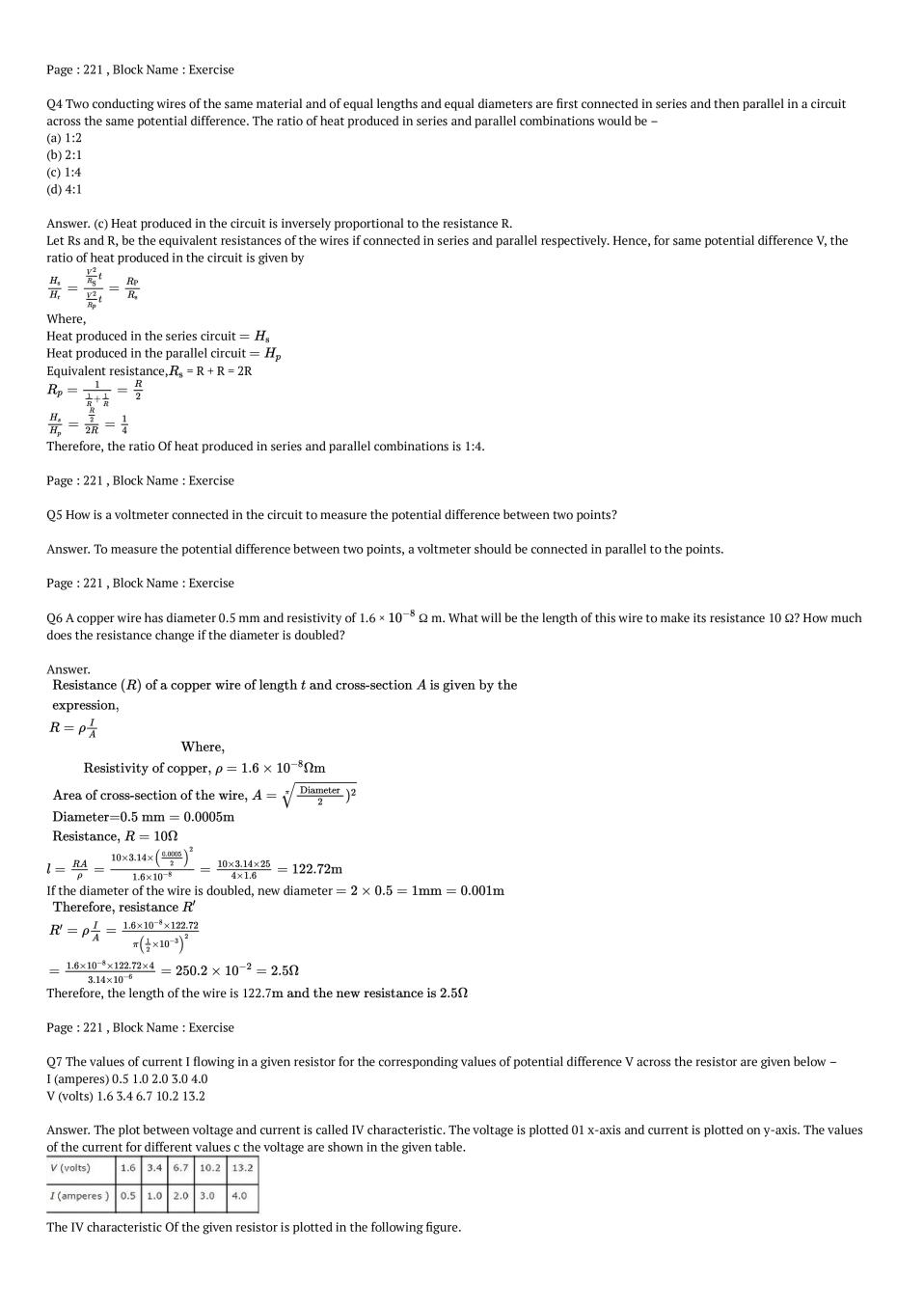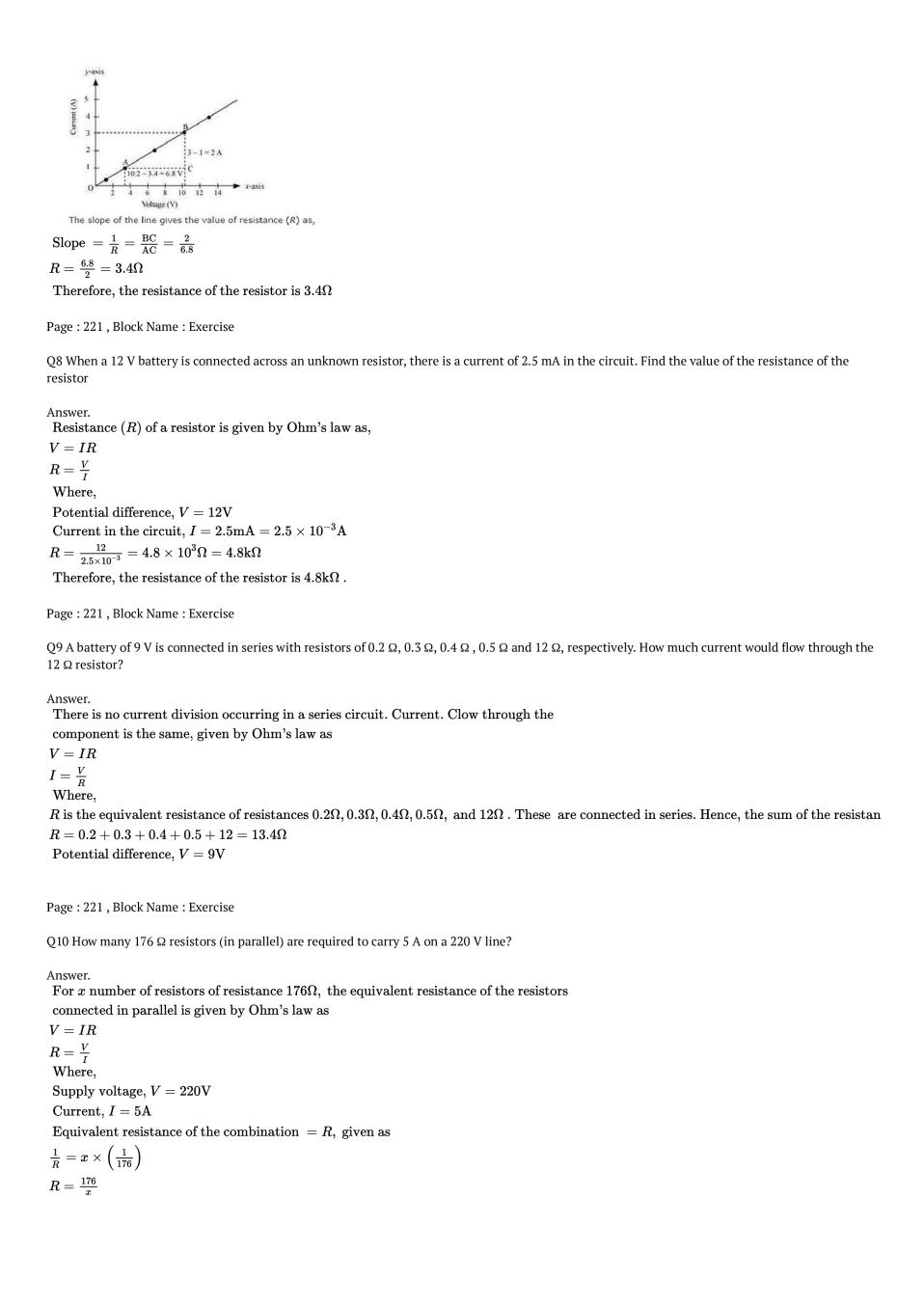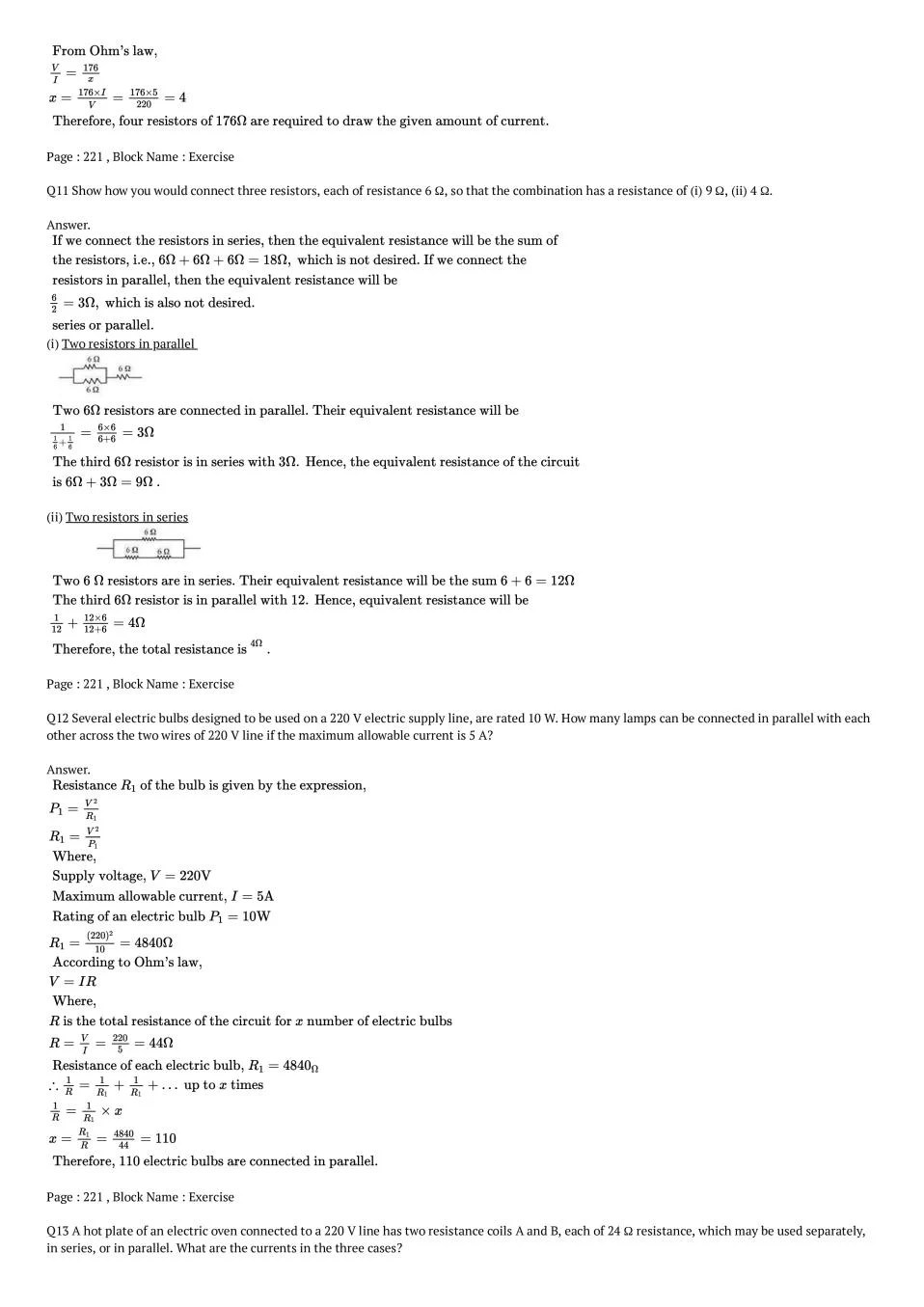### Class 10 Science Chapter 12 NCERT Solutions

Get step by step solutions for the questions given in class 10 Science textbook from the latest NCERT as per board exam guidelines.

### Class 10 Science Chapter 12 Important Topics

1. Electric Current and Circuit
2. Electric Potential and Potential Difference
3. Circuit Diagram
4. OHM’s Law
5. Factors on which the Resistance of a Conductor Depends
6. Resistance of a System of Resistors
–  Registers in Series
–  Registers in Parallel
7. Heating Effect of Electric Current
–  Practical Applications of Heating Effect of Electric Current
8. Electric Power

### Summary Chapter 12 Class 10 Science

• A stream of electrons moving through a conductor constitutes an electric current. Conventionally, the direction of current is taken opposite to the direction of flow of electrons.
• The SI unit of electric current is ampere.
• To set the electrons in motion in an electric circuit, we use a cell or a battery. A cell generates a potential difference across its terminals. It is measured in volts (V).
• Resistance is a property that resists the flow of electrons in a conductor. It controls the magnitude of the current. The SI unit of resistance is ohm (Ω).
• Ohm’s law: The potential difference across the ends of a resistor is directly proportional to the current through it, provided its temperature remains the same.
• The resistance of a conductor depends directly on its length, inversely on its area of cross-section, and also on the material of the conductor.
• The equivalent resistance of several resistors in series is equal to the sum of their individual resistances.
• A set of resistors connected in parallel has an equivalent resistance Rp given by, 1/Rp = (1/R1) + (1/R2) + (1/R3) + ……… + (1/Rn)
• The electrical energy dissipated in a resistor is given by: W = V × I × t
• The unit of power is watt (W). One watt of power is consumed when 1 A of current flows at a potential difference of 1 V.
• The commercial unit of electrical energy is kilowatt hour (kWh). {1 kWh = 3,600,000} {J = 3.6 × 10^6J}

Exercise Covered under Science Book Class 10 Science Chapter 12 NCERT Solutions

Here on this page we have covered following exercises from NCERT Science Book for Class 10 Science Chapter 12 Electricity

 Questions All Questions Solved Exercises 18 Questions with Solutions

We also suggest you to practice all the solved examples given in the NCERT book to clear your concepts on Electricity. You can buy NCERT Books for Class 10 Science online for doorstep delivery.

### NCERT Solutions Class 10 Chapter Wise

If you want NCERT solutions of any topic other than Chapter 12 Electricity, check it from here. Science Book by NCERT has all chapters that are in your Class 10 syllabus.

### NCERT Solutions Class 10 All Subjects

All NCERT Solutions for Subjects other than Science are provided below:

 English Hindi Science Maths Social Science

### Other Classes NCERT Solutions

If you want NCERT solutions of any class other than Class 10, check it from here.